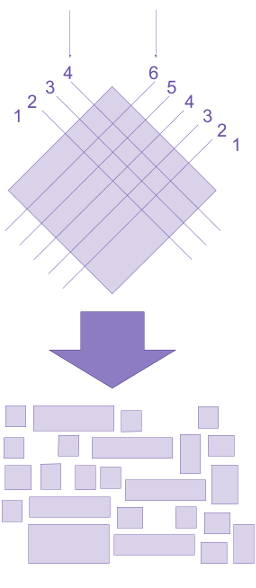# Commutativity: Examples

## Positive examples

$$x+y = y+x$$ for all numbers $$x$$ and $$y,$$ so addition commutes. One easy way to see this fact is to consider a physical system that implements addition, e.g., by taking two piles of poker chips (where a poker chip with nn chips represents the number nn) in on two input belts, and producing a pile of poker chips on the output belt. This function can be implemented by simply shoving both input piles onto the output pile. Because a pile of chips represents the same number regardless of how those chips are arranged on the output belt, it doesn’t matter which pile comes in on which input belt, so addition is commutative.

### Multiplication

$$x \times y = y \times x$$ for all numbers $$x$$ and $$y,$$ so multiplication also commutes. It is a bit harder to see why this must be so in the case of multiplication, as $$x \times y$$ can be interpreted as “take the $$x$$ input and stretch it out according to the $$y$$ input,” and it’s not entirely obvious that “stretching the $$x$$ input according to $$y$$” should yield the same result as “stretching the $$y$$ input according to $$x.$$

To see that multiplication commutes, it is helpful to visualize copying $$x$$ (rather than stretching it out) into $$y$$ many rows. This allows us to visualize $$x \times y$$ as a square divided into $$x$$ parts along one side and $$y$$ parts along the other side, which makes it clearer that multiplication commutes.It is worth noting that the concept of “multiplication” generalizes beyond the concept of “numbers,” and in those contexts, “stretching $$x$$ out according to $$y$$” and “stretching $$y$$ out according to $$x$$” might be quite different operations. For example, multiplication of matrices does not commute.

### Maximum and minimum

The max and min functions commute, because “which of these elements is largest/​smallest?” is a question that doesn’t depend upon the order that the elements are presented in.

### Rock, Paper, Scissors

The question of who won in a game of rock-paper-scissors is commutative. Writing $$r$$ for rock, $$p$$ for paper, $$s$$ for scissors, and $$?$$ for the binary operator saying who won, we have $$r ? p = p,$$ $$r ? s = r,$$ and $$p ? s = s,$$ and so on. This function is commutative: who won a game of rock-paper-scissors does not depend upon which side of the judge they were standing on.

This provides an example of a commutative function that is not associative: $$r?p=p?r$$ and so on, but $$(r?p)?s=s$$ while $$r?(p?s)=r.$$ In other words, who won a game of rock-paper-scissors does not depend on which side of the judge they were standing on, but if you have a line of people throw “rock,” “paper,” or “scissors,” then the question “who won?” does depend on which end of the line you start from.

## Negative examples

### Concatenation

Any function that includes its inputs in its outputs in order is not commutative. For example, the function pair which puts its inputs into a pair (such that pair(x, y) = (x, y)) is not commutative. The function concat, which also sticks its inputs together in order, is also not commutative, though it is associative.

### Division

Division is not commutative, because $$x / y$$ does not equal $$y / x$$ in general. In this case, the function is using $$x$$ and $$y$$ in a very different way. One is interpreted as a numerator that will be cut up, the other is treated as a denominator that says how many times to cut the numerator up. Numerator and denominator are not exchangeable concepts: Division needs to know which input is intended to be the numerator and which is intended to be the denominator.

### Matrix multiplication

Matrix multiplication is not only non-commutative, it may fail to even make sense. Two matrices can be multiplied if and only if the number of columns in the left-hand matrix is equal to the number of rows in the right-hand matrix. Thus, a $$2 \times 3$$ matrix can be multiplied with a $$3 \times 5$$ matrix if and only if the $$2 \times 3$$ matrix is on the left. Clearly, then, matrix multiplication does not commute.